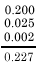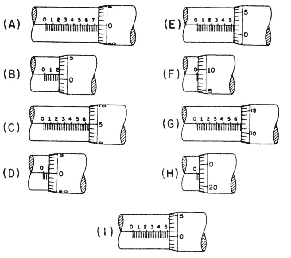Calculating with approximate numbersCustom SearchCALCULATING WITH APPROXIMATE NUMBERS The concepts of precision and accuracy form the basis for the rules which govern calculation with approximate numbers (numbers resulting from measurement). Addition and Subtraction A sum or difference can never be more precise than the least precise number in the calculation. Therefore, before adding or subtracting approximate numbers, they should be rounded to the same degree of precision. The more precise numbers are all rounded to the precision of the least precise number in the group to be combined. For example, the numbers 2.95, 32.7, and 1.414 would be rounded to tenths before adding as follows:Multiplication and Division When two numbers are multiplied, the result often has several more digits than either of the original factors. Division also frequently produces more digits in the quotient than the original data possessed, if the division is "carried out" to several decimal places. Results such as these appear to have more significant digits than the original measurements from which they came, giving the false impression of greater accuracy than is justified. In order to correct this situation, the following rule is used: In order to multiply or divide two approximate numbers having an equal number of significant digits, round the answer to the same number of significant digits as are shown in one of the original numbers. If one of the original factors has more significant digits than the other, round the more accurate number before multiplying. It should be rounded to one more significant digit than appears in the less accurate number; the extra digit protects the answer from the effects of multiple rounding. After performing the‘ multiplication or division, round the result to the same number of significant digits as are shown in the less accurate of the original factors. Practice problems: 1. Find the sum of the sides of a triangle in which the lengths of the three sides are as follows: 2.5 inches, 3.72 inches, and 4.996 inches. 2. Find the product of the length and width of a rectangle which is 2.95 feet long and 0.9046 foot wide. Answers: 1. 11.2 inches 2. 2.67 square feet MICROMETERS AND VERNIERS Closely associated with the study of decimals is a measuring instrument known as a micrometer. The ordinary micrometer is capable of measuring accurately to one -thousandth of an inch. One-thousandth of an inch is about the thickness of a human hair or a thin sheet of paper. The parts of a micrometer are shown in figure 6-l. MICROMETER SCALES The spindle and the thimble move together. The end of the spindle (hidden from view in figure 6-l) is a screw with 40 threads per inch. Consequently, one complete turn of the thimble moves the spindle one-fortieth of an inch orFigure 6-1.–(A) Parts of a micrometer; (B) micrometer scales. 0.025 inch since 1/40 is equal to 0.025. The sleeve has 40 markings to the inch. Thus each space between the markings on the sleeve is also 0.025 inch. Since 4 such spaces are 0.1 inch (that is, 4 x 0.025), every fourth mark is labeled in tenths of an inch for convenience in reading. Thus, 4 marks equal 0.1 inch, 8 marks equal 0.2 inch, 12 marks equal 0.3 inch, etc. To enable measurement of a partial turn, the beveled edge of the thimble is divided into 25 equal parts. Thus each marking on the thimble is 1/25 of a complete turn, or 1/25 of 1/40 of an inch. Multiplying 1/25 times 0.025 inch, we find that each marking on the thimble represents 0.001 inch. READING THE MICROMETER It is sometimes convenient when learning to read a micrometer to write down the component parts of the measurement as read on the scales and then to add them. For example, in figure 6-1 (B) there are two major divisions visible (0.2 inch). One minor division is showing clearly (0.025 inch). The marking on the thimble nearest the horizontal or index line of the sleeve is the second marking (0.002 inch). Adding these parts, we haveThus, the reading is 0.227 inch. As explained previously, this is read verbally as "two hundred twenty-seven thousandths." A more skillful method of reading the scales is to read all digits as thousandths directly and to do any adding mentally. Thus, we read the major division on the scale as "two hundred thousandths" and the minor division is added on mentally. The mental process for the above setting then would be "two hundred twenty-five; two hundred twenty-seven thousandths." Practice problems: 1. Read each of the micrometer settings shown in figure 6-2.Figure 6-2.–Micrometer settings. Answers: (A) 0.750 (B) 0.201  (C) 0.655  (D) 0.075  (E) 0.527 (F) 0.009 (G) 0.662 (H) 0.048 (I) 0.526Integrated Publishing, Inc. - A (SDVOSB) Service Disabled Veteran Owned Small Business